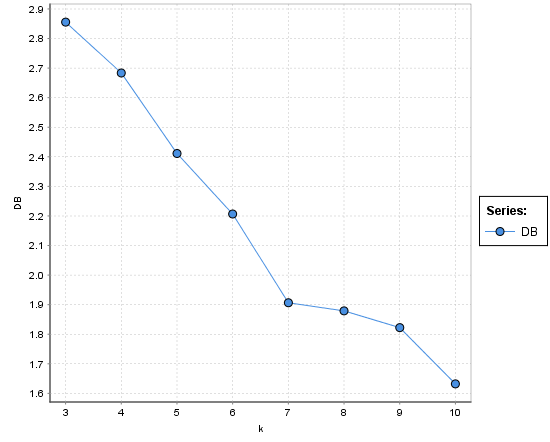# choose best cluster number

Member Posts: 2Contributor I
edited November 2018 in HelpHi

I have this chart for find best cluster number based on davies bouldin index and kmeans algorithm....i don't have local minimum in this chart, should I choose 7 cluster?? why ??? what should we do when we don't have local minimum?

Tagged:

• Moderator, RapidMiner Certified Analyst, RapidMiner Certified Expert, Member Posts: 1,635Unicorn
Solution Accepted

With high dimensional data, it can be hard to know what the "best" number of clusters is and visual inspection of the data usually does not work.  Unless you have an a priori preference for a specific number, you often will look for the tradeoffs between adding additional clusters and the marginal improvement in some global fitness metric (like the DB index), which is often referred to as the "elbow method" of cluster selection, as described here: https://en.wikipedia.org/wiki/Elbow_method_(clustering)

Based on that logic, I would probably select k=7 from your results, since the benefit of adding additional clusters is minimal (and thus there is a significant inflection point and change in slope at that point in the graph).

Brian T.
Lindon Ventures
Data Science Consulting from Certified RapidMiner Experts

• Moderator, RapidMiner Certified Analyst, Member Posts: 1,195Unicorn

Hi @shiva1,

Maybe a first step, is to perform an Exploratory Data Analysis to determine visually how many cluster there are. (you

go to the Charts panels and you can represent graphically your data.

A second approach is to use the DBSCAN operator (an other clustering method) who does not need

to have the number of cluster k as entry parameter.

I hope this first response elements will be useful.

Regards,

Lionel

• Moderator, RapidMiner Certified Analyst, Member Posts: 1,195Unicorn

Hi @shiva1,

To estimate the right number of k, we can use the Bayesian Information Criterion (BIC).

I have tested an algorithm based on this criterion on the well known dataset "Iris" which contains 3 class :

The algorithms conclude that the right number of clusters was 3, so I think it can be relevant.

So I propose to you, to share your dataset in order to execute this algorithm on your dataset

Regards and happy new year 2018 !

Lionel

• Member Posts: 2Contributor I

thanks

but i have text data and dbscan is not a good choice for text mining...cause it usually turn only one cluster

• Member Posts: 73Contributor II

Hello. Excuse me a question that has engaged my mind
If in the operator performance by distance
Choose the maximaization option
In this case, according to the first post chart
k = 3 is the best value?
That is better db with high value?
Thank you for asking me questions

• Administrator, Employee, RapidMiner Certified Analyst, RapidMiner Certified Expert, Member Posts: 363RM Data Scientist

"clustering algorithm that produces a collection of clusters with the smallest Davies–Bouldin index is considered the best algorithm" -Wikipedia.

The Davies-Bouldin Index evaluates intra-cluster similarity and inter-cluster differences. If you consider these to be good criteria, go for the Davies-Bouldin.

My attached process is an optimization to pick the best K for K-means model, which returns k=3 has the lowest D-B index. You can also try X-mean to get an optimized clustering.

The D-B index was multiplied by -1 internally for maximizing it. You could ignore the negative sign from the performance output.

`<?xml version="1.0" encoding="UTF-8"?><process version="8.2.001">  <context>    <input/>    <output/>    <macros/>  </context>  <operator activated="true" class="process" compatibility="6.0.002" expanded="true" name="Process">    <parameter key="notification_email" value="[email protected]"/>    <parameter key="process_duration_for_mail" value="1"/>    <parameter key="encoding" value="UTF-8"/>    <process expanded="true">      <operator activated="true" class="retrieve" compatibility="8.2.001" expanded="true" height="68" name="Ripley-Set" width="90" x="45" y="34">        <parameter key="repository_entry" value="//Samples/data/Ripley-Set"/>      </operator>      <operator activated="true" class="multiply" compatibility="8.2.001" expanded="true" height="103" name="Multiply" width="90" x="279" y="34"/>      <operator activated="true" class="concurrency:optimize_parameters_grid" compatibility="8.2.000" expanded="true" height="145" name="Optimize Parameters" width="90" x="514" y="34">        <list key="parameters">          <parameter key="Clustering.k" value="[2.0;20;19;linear]"/>        </list>        <process expanded="true">          <operator activated="true" class="fast_k_means" compatibility="8.2.001" expanded="true" height="82" name="Clustering" width="90" x="246" y="34"/>          <operator activated="true" class="cluster_distance_performance" compatibility="8.2.001" expanded="true" height="103" name="Performance" width="90" x="648" y="34">            <parameter key="main_criterion" value="Davies Bouldin"/>            <parameter key="main_criterion_only" value="true"/>          </operator>          <connect from_port="input 1" to_op="Clustering" to_port="example set"/>          <connect from_op="Clustering" from_port="cluster model" to_op="Performance" to_port="cluster model"/>          <connect from_op="Clustering" from_port="clustered set" to_op="Performance" to_port="example set"/>          <connect from_op="Performance" from_port="performance" to_port="performance"/>          <connect from_op="Performance" from_port="example set" to_port="output 1"/>          <connect from_op="Performance" from_port="cluster model" to_port="model"/>          <portSpacing port="source_input 1" spacing="0"/>          <portSpacing port="source_input 2" spacing="0"/>          <portSpacing port="sink_performance" spacing="0"/>          <portSpacing port="sink_model" spacing="0"/>          <portSpacing port="sink_output 1" spacing="0"/>          <portSpacing port="sink_output 2" spacing="0"/>          <description align="left" color="green" colored="true" height="173" resized="false" width="626" x="109" y="164">Davies-Bouldin Index evaluates intra-cluster similarity and inter-cluster differences. If you consider these to be good criteria, go for the Davies-Bouldin. The Silhouette Index measure the distance between each data point, the centroid of the cluster it was assigned to and the closest centroid belonging to another cluster. If you consider that this is a good criterion, go for the silhouette index.&lt;br&gt;&lt;br&gt;How can we say that a clustering quality measure is good?. Available from: https://www.researchgate.net/post/How_can_we_say_that_a_clustering_quality_measure_is_good.</description&gt;        </process>        <description align="center" color="transparent" colored="false" width="126">figure out the best k for k-means</description>      </operator>      <operator activated="true" class="x_means" compatibility="8.2.001" expanded="true" height="82" name="X-Means" width="90" x="514" y="289">        <parameter key="k_max" value="10"/>        <description align="center" color="transparent" colored="false" width="126">run x-means for an optimzied clustering</description>      </operator>      <connect from_op="Ripley-Set" from_port="output" to_op="Multiply" to_port="input"/>      <connect from_op="Multiply" from_port="output 1" to_op="Optimize Parameters" to_port="input 1"/>      <connect from_op="Multiply" from_port="output 2" to_op="X-Means" to_port="example set"/>      <connect from_op="Optimize Parameters" from_port="parameter set" to_port="result 1"/>      <connect from_op="Optimize Parameters" from_port="output 1" to_port="result 2"/>      <connect from_op="X-Means" from_port="clustered set" to_port="result 3"/>      <portSpacing port="source_input 1" spacing="0"/>      <portSpacing port="sink_result 1" spacing="42"/>      <portSpacing port="sink_result 2" spacing="0"/>      <portSpacing port="sink_result 3" spacing="189"/>      <portSpacing port="sink_result 4" spacing="0"/>    </process>  </operator></process>`
• Member Posts: 93Maven
Hi @shiva1

why is DBSCAN not a good option to apply on text data?